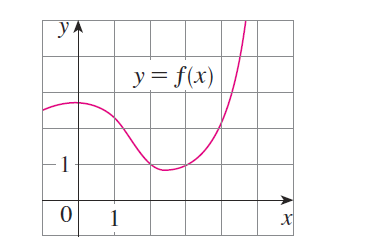#### Problem 66E

66. If $f$ is the function whose graph is shown, let $h(x)=f(f(x))$ and $g(x)=f\left(x^{2}\right)$. Use the graph of $f$ to estimate the value of each derivative.

(a) $h^{\prime}(2)$

(b) $g^{\prime}(2)$